CBSE (Science) Class 11CBSE
Share

# HC Verma solutions for Class 11 Physics chapter 5 - Specific Heat Capacities of Gases

#### HC Verma Concepts of Physics - Vol. 2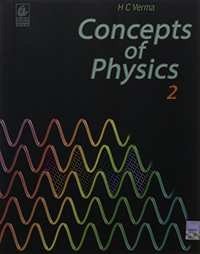## Chapter 5: Specific Heat Capacities of Gases

OthersMCQ

#### HC Verma solutions for Class 11 Physics Chapter 5 [Page 76]

Q 1 | Page 76

Does a gas have just two specific heat capacities or more than two? Is the number of specific heat capacities of a gas countable?

Q 2 | Page 76

Can we define specific heat capacity at constant temperature?

Q 3 | Page 76

Can we define specific heat capacity for an adiabatic process?

Q 4 | Page 76

Does a solid also have two kinds of molar heat capacities Cp and Cv? If yes, is Cp > Cv? Or is Cp − Cv = R?

Q 5 | Page 76

In a real gas, the internal energy depends on temperature and also on volume. The energy increases when the gas expands isothermally. Examining the derivation of Cp − Cv = R, find whether Cp − Cv will be more than R, less than R or equal to R for a real gas.

Q 6 | Page 76

Can a process on an ideal gas be both adiabatic and isothermal?

Q 7 | Page 76

Show that the slope of the p−V diagram is greater for an adiabatic process compared to an isothermal process.

Q 8 | Page 76

Is a slow process always isothermal? Is a quick process always adiabatic?

Q 9 | Page 76

Can two states of an ideal gas be connected by an isothermal process as well as an adiabatic process?

Q 10 | Page 76

The ratio Cp / Cv for a gas is 1.29. What is the degree of freedom of the molecules of this gas?

#### HC Verma solutions for Class 11 Physics Chapter 5 Exercise MCQ [Pages 76 - 77]

MCQ | Q 1 | Page 76

Work done by a sample of an ideal gas in a process A is double the work done in another process B. The temperature rises through the same amount in the two processes. If CAand CB be the molar heat capacities for the two processes,

• CA = CB

• CA < CB

• CA > CB

•  CA and CB cannot be defined.

MCQ | Q 2 | Page 76

For a solid with a small expansion coefficient,

• Cp − Cv = R

• Cp = Cv

•  Cp is slightly greater than Cv

•  Cp is slightly less than Cv

MCQ | Q 3 | Page 76

The value of Cp − Cv is 1.00 R for a gas sample in state A and 1.08 R in state B. Let pAand pB denote the pressures and TA and TB denote the temperatures of the states A and B, respectively. It is most likely that

•  pA < pB and TA > TB

•  pA > pB and TA < TB

• pA = pB and TA < TB

• pA > pB and TA = TB

MCQ | Q 4 | Page 76

Let Cv and Cp denote the molar heat capacities of an ideal gas at constant volume and constant pressure respectively. Which of the following is a universal constant?

• ("C"_"P")/("C"_"P")

• CpCv

• Cp − Cv

•  Cp + Cv

MCQ | Q 5 | Page 76

70 calories of heat are required to raise the temperature of 2 mole of an ideal gas at constant pressure from 30° C to 35° C. The amount of heat required to raise the temperature of the same gas through the same range at constant volume is

• 30 calories

• 50 calories

• 70 calories

• 90 calories

MCQ | Q 6 | Page 76

The figure shows a process on a gas in which pressure and volume both change. The molar heat capacity for this process is C.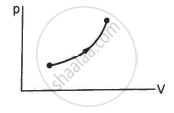• C = 0

•  C = Cv

• C > Cv

•  C < Cv

MCQ | Q 7 | Page 76

The molar heat capacity for the process shown in the figure is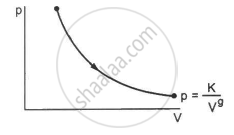•  C = Cp

•  C = Cv

•  C > Cv

•  C = 0.

MCQ | Q 8 | Page 76

In an isothermal process on an ideal gas, the pressure increases by 0.5%. The volume decreases by about

• 0.25%

• 0.5%

•  0.7%

• 1%.

MCQ | Q 9 | Page 76

In an adiabatic process on a gas with γ = 1.4, the pressure is increased by 0.5%. The volume decreases by about

• 0.36%

• 0.5%

•  0.7%

• 1%

MCQ | Q 10 | Page 76

Two samples A and B are initially kept in the same state. Sample A is expanded through an adiabatic process and the sample B through an isothermal process. The final volumes of the samples are the same. The final pressures in A and B are pA and pBrespectively.

• pA > pB

•  pA = pB

• pA < pB

• The relation between pA and pB cannot be deduced.

MCQ | Q 11 | Page 76

Let Ta and Tb be the final temperatures of the samples A and B, respectively, in the previous question.

• Ta < Tb

• Ta = Tb

• Ta > Tb

• The relation between Ta and Tb cannot be deduced.

MCQ | Q 12 | Page 76

Let ∆Wa and ∆Wb be the work done by the systems A and B, respectively, in the previous question.

• ∆Wa > ∆Wb

•  ∆Wa = ∆Wb

• ∆Wa < ∆Wb

• The relation between ∆Wa and ∆Wb cannot be deduced.

MCQ | Q 13 | Page 77

The molar heat capacity of oxygen gas at STP is nearly 2.5 R. As the temperature is increased, it gradually increases and approaches 3.5 R. The most appropriate reason for this behaviour is that at high temperatures

•  oxygen does not behave as an ideal gas

• oxygen molecules dissociate in atoms

•  the molecules collide more frequently

• molecular vibrations gradually become effective

#### HC Verma solutions for Class 11 Physics Chapter 5 Exercise MCQ [Page 77]

Q 1 | Page 77

A gas kept in a container of finite conductivity is suddenly compressed. The process
(a) must be very nearly adiabatic
(b) must be very nearly isothermal
(c) may be very nearly adiabatic
(d) may be very nearly isothermal

MCQ | Q 2 | Page 77

Let Q and W denote the amount of heat given to an ideal gas and the work done by it in an isothermal process.

• Q = 0

•  W = 0

• Q ≠ W

• Q = W

Q 3 | Page 77

Let Q and W denote the amount of heat given to an ideal gas and the work done by it in an adiabatic process.
(a) Q = 0
(b) W = 0
(c) Q = W
(d) Q ≠ W

MCQ | Q 4 | Page 77

Consider the processes A and B shown in the figure. It is possible that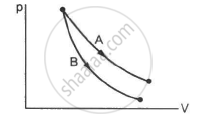• both the processes are isothermal

• both the processes are adiabatic

• A is isothermal and B is adiabatic

• A is adiabatic and B is isothermal

Q 5 | Page 77

Three identical adiabatic containers A, B and C contain helium, neon and oxygen, respectively, at equal pressure. The gases are pushed to half their original volumes.
(a) The final temperatures in the three containers will be the same.
(b) The final pressures in the three containers will be the same.
(c) The pressures of helium and neon will be the same but that of oxygen will be different.
(d) The temperatures of helium and neon will be the same but that of oxygen will be different.

Q 6 | Page 77

A rigid container of negligible heat capacity contains one mole of an ideal gas. The temperature of the gas increases by 1° C if 3.0 cal of heat is added to it. The gas may be
(a) helium
(b) argon
(c) oxygen
(d) carbon dioxide

MCQ | Q 7 | Page 77

Four cylinders contain equal number of moles of argon, hydrogen, nitrogen and carbon dioxide at the same temperature. The energy is minimum in

• argon

• hydrogen

• nitrogen

• carbon dioxide

#### HC Verma solutions for Class 11 Physics Chapter 5 [Pages 77 - 80]

Q 1 | Page 77

A vessel containing one mole of a monatomic ideal gas (molecular weight = 20 g mol−1) is moving on a floor at a speed of 50 m s−1. The vessel is stopped suddenly. Assuming that the mechanical energy lost has gone into the internal energy of the gas, find the rise in its temperature.

Q 2 | Page 77

5 g of a gas is contained in a rigid container and is heated from 15°C to 25°C. Specific heat capacity of the gas at constant volume is 0.172 cal g−1 °C−1 and the mechanical equivalent of heat is 4.2 J cal−1. Calculate the change in the internal energy of the gas

Q 3 | Page 77

The figure shows a cylindrical container containing oxygen (γ = 1.4) and closed by a 50-kg frictionless piston. The area of cross-section is 100 cm2, atmospheric pressure is 100 kPa and g is 10 m s−2. The cylinder is slowly heated for some time. Find the amount of heat supplied to the gas if the piston moves out through a distance of 20 cm.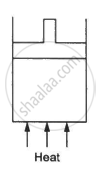Q 4 | Page 77

The specific heat capacities of hydrogen at constant volume and at constant pressure are 2.4 cal g−1 °C−1 and 3.4 cal g−1 °C−1 respectively. The molecular weight of hydrogen is 2 g mol−1 and the gas constant, R = 8.3 × 107 erg °C−1 mol−1. Calculate the value of J.

Q 5 | Page 77

The ratio of the molar heat capacities of an ideal gas is Cp/Cv = 7/6. Calculate the change in internal energy of 1.0 mole of the gas when its temperature is raised by 50 K (a) keeping the pressure constant (b) keeping the volume constant and (c) adiaba

Q 6 | Page 77

A sample of air weighing 1.18 g occupies 1.0 × 103 cm3 when kept at 300 K and 1.0 × 105 Pa. When 2.0 cal of heat is added to it at constant volume, its temperature increases by 1°C. Calculate the amount of heat needed to increase the temperature of air by 1°C at constant pressure if the mechanical equivalent of heat is  4.2 × 107 erg cal−1. Assume that air behaves as an ideal gas.

Q 7 | Page 78

An ideal gas expands from 100 cm3 to 200 cm3 at a constant pressure of 2.0 × 105 Pa when 50 J of heat is supplied to it. Calculate (a) the change in internal energy of the gas (b) the number of moles in the gas if the initial temperature is 300 K (c) the molar heat capacity Cp at constant pressure and (d) the molar heat capacity Cv at constant volume.

Q 8 | Page 78

An amount Q of heat is added to a monatomic ideal gas in a process in which the gas performs a work Q/2 on its surrounding. Find the molar heat capacity for the process

Q 9 | Page 78

An ideal gas is taken through a process in which the pressure and the volume are changed according to the equation p = kV. Show that the molar heat capacity of the gas for the process is given by "C" ="C"_"v" +"R"/2.

Q 10 | Page 78

An ideal gas (Cp / Cv = γ) is taken through a process in which the pressure and the volume vary as p = aVb. Find the value of b for which the specific heat capacity in the process is zero.

Q 11 | Page 78

Two ideal gases have the same value of Cp / Cv = γ. What will be the value of this ratio for a mixture of the two gases in the ratio 1 : 2?

Q 12 | Page 78

A mixture  contains 1 mole of helium (Cp = 2.5 R, Cv = 1.5 R) and 1 mole of hydrogen (Cp= 3.5 R, Cv = 2.5 R). Calculate the values of Cp, Cv and γ for the mixture.

Q 13 | Page 78

Half mole of an ideal gas (γ = 5/3) is taken through the cycle abcda, as shown in the figure. Take  "R" = 25/3"J""K"^-1 "mol"^-1 . (a) Find the temperature of the gas in the states a, b, c and d. (b) Find the amount of heat supplied in the processes ab and bc. (c) Find the amount of heat liberated in the processes cd and da.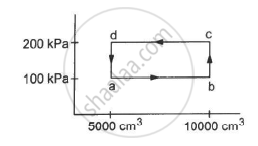Q 14 | Page 78

An ideal gas (γ = 1.67) is taken through the process abc shown in the figure. The temperature at point a is 300 K. Calculate (a) the temperatures at b and c (b) the work done in the process (c) the amount of heat supplied in the path ab and in the path bcand (d) the change in the internal energy of the gas in the process.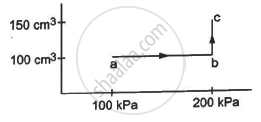Q 15 | Page 78

In Joly's differential steam calorimeter, 3 g of an ideal gas is contained in a rigid closed sphere at 20°C. The sphere is heated by steam at 100°C and it is found that an extra 0.095 g of steam has condensed into water as the temperature of the gas becomes constant. Calculate the specific heat capacity of the gas in J g−1 K−1. The latent heat of vaporisation of water = 540 cal g−1

Q 16 | Page 78

The volume of an ideal gas (γ = 1.5) is changed adiabatically from 4.00 litres to 3.00 litres. Find the ratio of (a) the final pressure to the initial pressure and (b) the final temperature to the initial temperature.

Q 17 | Page 78

An ideal gas at pressure 2.5 × 105 Pa and temperature 300 K occupies 100 cc. It is adiabatically compressed to half its original volume. Calculate (a) the final pressure (b) the final temperature and (c) the work done by the gas in the process. Take γ = 1.5

Q 18 | Page 78

Air (γ = 1.4) is pumped at 2 atm pressure in a motor tyre at 20°C. If the tyre suddenly bursts, what would be the temperature of the air coming out of the tyre? Neglect any mixing with the atmospheric air.

Q 19 | Page 78

A gas is enclosed in a cylindrical can fitted with a piston. The walls of the can and the piston are adiabatic. The initial pressure, volume and temperature of the gas are 100 kPa, 400 cm3 and 300 K, respectively. The ratio of the specific heat capacities of  the gas, Cp / Cv = 1.5. Find the pressure and the temperature of the gas if it is (a) suddenly compressed (b) slowly compressed to 100 cm3.

Q 20 | Page 78

The initial pressure and volume of a given mass of a gas (Cp/Cv = γ) are p0 and V0. The gas can exchange heat with the surrounding. (a) It is slowly compressed to a volume V0/2 and then suddenly compressed to V0/4. Find the final pressure. (b) If the gas is suddenly compressed from the volume V0 to V0/2 and then slowly compressed to V0/4, what will be the final pressure?

Q 21 | Page 78

Consider a given sample of an ideal gas (Cp/Cv = γ) having initial pressure p0 and volume V0. (a) The gas is  isothermally taken to a pressure p0/2 and from there, adiabatically to a pressure p0/4. Find the final volume. (b) The gas is brought back to its initial state. It is adiabatically taken to a pressure p0/2 and from there, isothermally to a pressure p0/4. Find the final volume.

Q 22 | Page 78

A sample of an ideal gas (γ = 1.5) is compressed adiabatically from a volume of 150 cm3 to 50 cm3. The initial pressure and the initial temperature are 150 kPa and 300 K. Find (a) the number of moles of the gas in the sample (b) the molar heat capacity at constant volume (c) the final pressure and temperature (d) the work done by the gas in the process and (e) the change in internal energy of the gas.

Q 23 | Page 79

Three samples A, B and C of the same gas (γ = 1.5) have equal volumes and temperatures. The volume of each sample is doubled, the process being isothermal for A, adiabatic for B and isobaric for C. If the final pressures are equal for the three samples, find the ratio of the initial pressures.

Q 24 | Page 79

Two samples A and B, of the same gas have equal volumes and pressures. The gas in sample A is expanded isothermally to double its volume and the gas in B is expanded adiabatically to double its volume. If the work done by the gas is the same for the two cases, show that γ satisfies the equation 1 − 21−γ = (γ − 1) ln2.

Q 25 | Page 79

1 litre of an ideal gas (γ = 1.5) at 300 K is suddenly compressed to half its original volume. (a) Find the ratio of the final pressure to the initial pressure. (b) If the original pressure is 100 kPa, find the work done by the gas in the process. (c) What is the change in internal energy? (d) What is the final temperature? (e) The gas is now cooled to 300 K keeping its pressure constant. Calculate the work done during the process. (f) The gas is now expanded isothermally to achieve its original volume of 1 litre. Calculate the work done by the gas. (g) Calculate the total work done in the cycle.

Q 26 | Page 79

Figure shows a cylindrical tube with adiabatic walls and fitted with an adiabatic separator. The separator can be slid into the tube by an external mechanism. An ideal gas (γ = 1.5) is injected in the two sides at equal pressures and temperatures. The separator remains in equilibrium at the middle. It is now slid to a position where it divides the tube in the ratio 1 : 3. Find the ratio of the temperatures in the two parts of the vessel.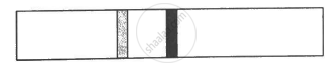Q 27 | Page 79

Figure shows two rigid vessels A and B, each of volume 200 cm3, containing an ideal gas (Cv = 12.5 J K−1 mol−1). The vessels are connected to a manometer tube containing mercury. The pressure in both the vessels is 75 cm of mercury and the temperature is 300 K. (a) Find the number of moles of the gas in each vessel. (b) 5.0 J of heat is supplied to the gas in vessel A and 10 J to the gas in vessel B. Assuming there's no appreciable transfer of heat from A to B, calculate the difference in the heights of mercury in the two sides of the manometer. Gas constant, R = 8.3 J K−1 mol−1.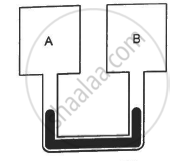Q 28 | Page 79

The figure shows two vessels with adiabatic walls, one containing 0.1 g of helium (γ = 1.67, M = 4 g mol−1)  and the other containing some amount of hydrogen (γ = 1.4, M = 2 g mol−1). Initially, the temperatures of the two gases are equal. The gases are electrically heated for some time during which equal amounts of heat are given to the two gases. It is found that the temperatures rise through the same amount in the two vessels. Calculate the mass of hydrogen.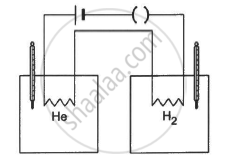Q 29 | Page 79

Two vessels A and B of equal volume V0 are connected by a narrow tube that can be closed by a valve. The vessels are fitted with pistons that can be moved to change the volumes. Initially, the valve is open and the vessels contain an ideal gas (Cp/Cv = γ) at atmospheric pressure p0 and atmospheric temperature T0. The walls of vessel A are diathermic and those of B are adiabatic. The valve is now closed and the pistons are slowly pulled out to increase the volumes of the vessels to double the original value. (a) Find the temperatures and pressures in the two vessels. (b) The valve is now opened for sufficient time so that the gases acquire a common temperature and pressure. Find the new values of the temperature and pressure.

Q 30 | Page 79

The figure shows an adiabatic cylindrical tube of volume V0 divided in two parts by a frictionless adiabatic separator. Initially, the separator is kept in the middle, an ideal gas at pressure p1 and temperature T1 is injected into the left part and another ideal gas at pressure p2 and temperature T2 is injected into the right part. Cp/Cv = γ is the same for both the gases. The separator is slid slowly and is released at a position where it can stay in equilibrium. Find (a) the volumes of the two parts (b) the heat given to the gas in the left part and (c) the final common pressure of the gases.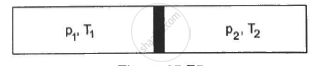Q 31 | Page 79

An adiabatic cylindrical tube of cross-sectional area 1 cm2 is closed at one end and fitted with a piston at the other end. The tube contains 0.03 g of an ideal gas. At 1 atm pressure and at the temperature of the surrounding, the length of the gas column is 40 cm. The piston is suddenly pulled out to double the length of the column. The pressure of the gas falls to 0.355 atm. Find the speed of sound in the gas at atmospheric temperature.

Q 32 | Page 80

The speed of sound in hydrogen at 0°C is 1280 m s−1. The density of hydrogen at STP is 0.089 kg m−3. Calculate the molar heat capacities Cp and Cv of hydrogen.

Q 33 | Page 80

4.0 g of helium occupies 22400 cm3 at STP. The specific heat capacity of helium at constant pressure is 5.0 cal K−1 mol−1. Calculate the speed of sound in helium at STP.

Q 34 | Page 80

An ideal gas of density 1.7 × 10−3 g cm−3 at a pressure of 1.5 × 105 Pa is filled in a Kundt's tube. When the gas is resonated at a frequency of 3.0 kHz, nodes are formed at a separation of 6.0 cm. Calculate the molar heat capacities Cp and Cv of the gas.

Q 35 | Page 80

Standing waves of frequency 5.0 kHz are produced in a tube filled with oxygen at 300 K. The separation between the consecutive nodes is 3.3 cm. Calculate the specific heat capacities Cp and Cv of the gas.

## Chapter 5: Specific Heat Capacities of Gases

OthersMCQ

#### HC Verma Concepts of Physics - Vol. 2## HC Verma solutions for Class 11 Physics chapter 5 - Specific Heat Capacities of Gases

HC Verma solutions for Class 11 Physics chapter 5 (Specific Heat Capacities of Gases) include all questions with solution and detail explanation. This will clear students doubts about any question and improve application skills while preparing for board exams. The detailed, step-by-step solutions will help you understand the concepts better and clear your confusions, if any. Shaalaa.com has the CBSE Concepts of Physics - Vol. 2 solutions in a manner that help students grasp basic concepts better and faster.

Further, we at Shaalaa.com provide such solutions so that students can prepare for written exams. HC Verma textbook solutions can be a core help for self-study and acts as a perfect self-help guidance for students.

Concepts covered in Class 11 Physics chapter 5 Specific Heat Capacities of Gases are Specific Heat Capacities - Gases, Mean Free Path, Kinetic Theory of Gases - Concept of Pressure, Behaviour of Gases, Equation of State of a Perfect Gas, Work Done in Compressing a Gas, Introduction of Kinetic Theory of an Ideal Gas, Kinetic Interpretation of Temperature, Kinetic Theory of Gases- Assumptions, rms Speed of Gas Molecules, Degrees of Freedom, Avogadro's Number, Molecular Nature of Matter, Law of Equipartition of Energy.

Using HC Verma Class 11 solutions Specific Heat Capacities of Gases exercise by students are an easy way to prepare for the exams, as they involve solutions arranged chapter-wise also page wise. The questions involved in HC Verma Solutions are important questions that can be asked in the final exam. Maximum students of CBSE Class 11 prefer HC Verma Textbook Solutions to score more in exam.

Get the free view of chapter 5 Specific Heat Capacities of Gases Class 11 extra questions for Physics and can use Shaalaa.com to keep it handy for your exam preparation

S